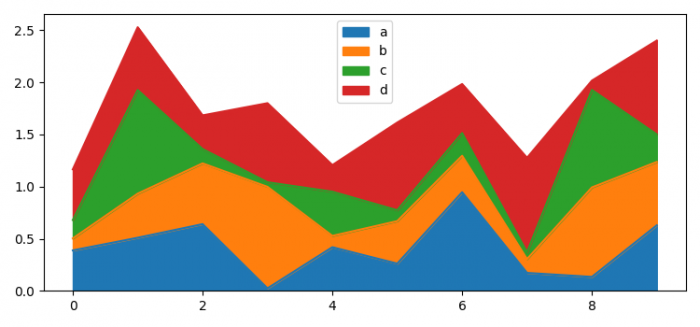# How to plot an area in a Pandas dataframe in Matplotlib Python?

To plot an area in a Pandas dataframe in Matplotlib Python, we can take the following steps −

• Set the figure size and adjust the padding between and around the subplots.
• Create a Pandas dataframe, i.e., a two-dimensional, size-mutable, potentially heterogeneous tabular data.
• Return the area between the graph plots.
• To display the figure, use show() method.

## Example

import pandas as pd
import numpy as np

from matplotlib import pyplot as plt

plt.rcParams["figure.figsize"] = [7.50, 3.50]
plt.rcParams["figure.autolayout"] = True

df = pd.DataFrame(np.random.rand(10, 4),
columns=["a", "b", "c", "d"])

df.plot.area()

plt.show()

## Output